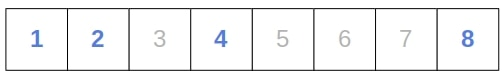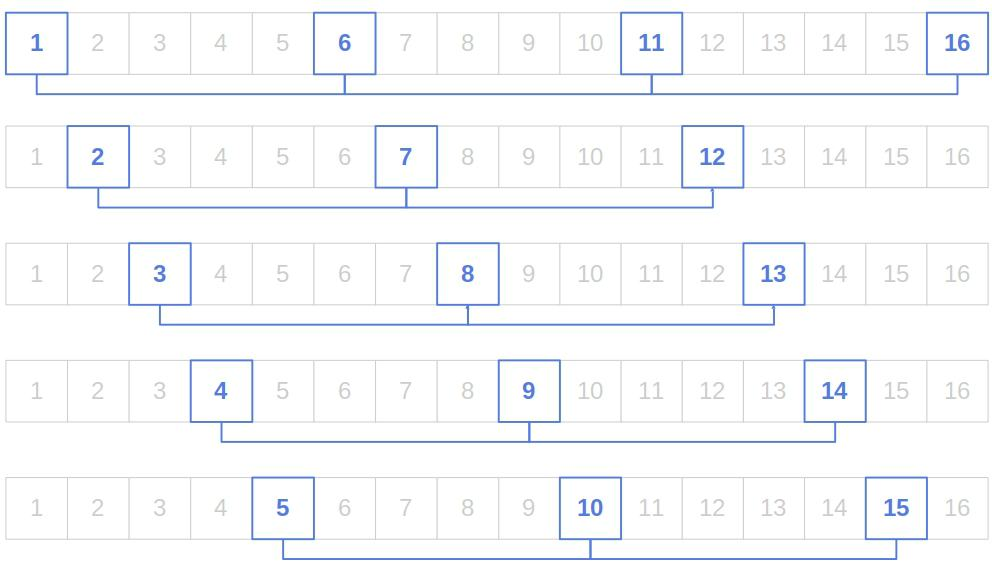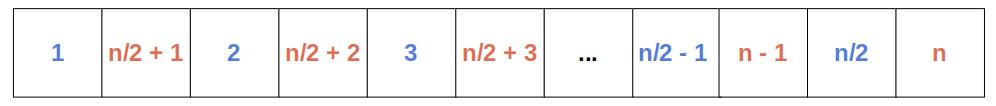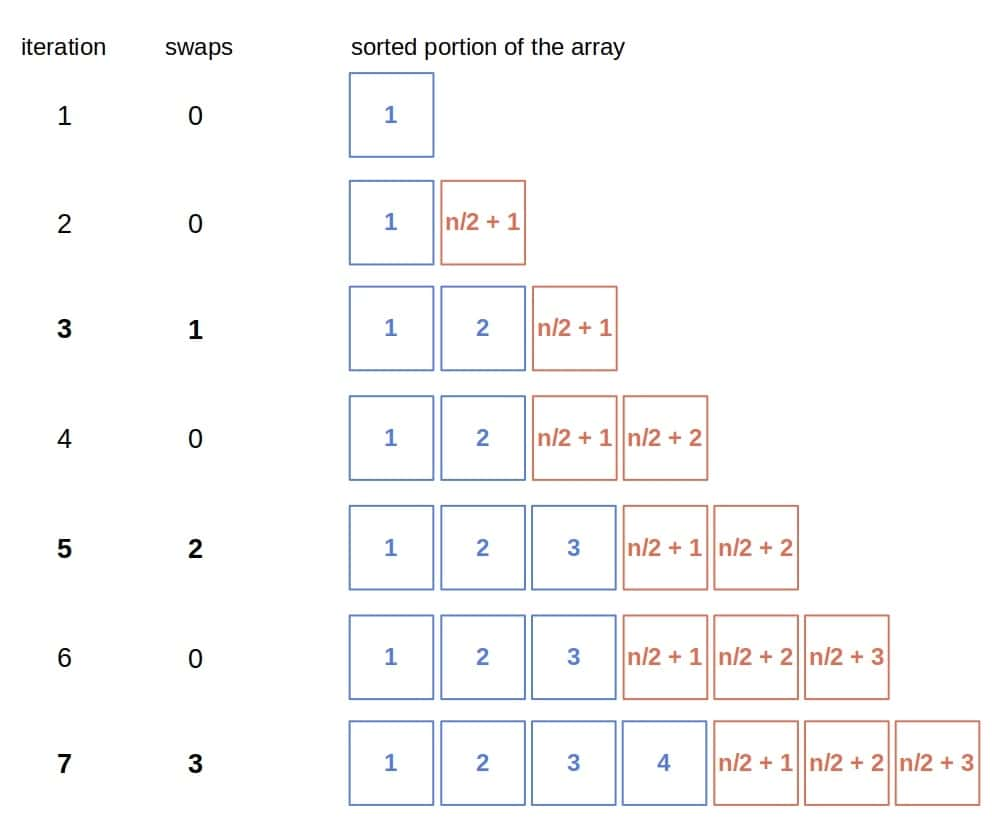## 1. Introduction

In this tutorial, we’ll talk about the complexity of Shellsort.

## 2. Shellsort

The algorithm uses the following property of arrays. Every subsequence of a sorted array is also sorted.

So, ifis a sorted array, however we choose indices, it will hold that. For instance:Shellsort considers the subsequences in which there’s a constant gap between the elements. For example, ifhas 16 elements, and the gap is 4, we have five subsequences or chains, as we may call them:In each pass, Shellsort sorts individual chains using Insertion Sort, reduces the gap, and sorts the new chains in the subsequent pass. The rationale is that by sorting the chains with large gaps, the elements may “jump” over large portions of the array, so there will be less work when sorting chains with smaller gaps. Additionally, by reducing the gaps over time, the chains get larger and include more indices, so they place the elements closer to their correct positions.

### 2.1. Pseudocode

Here’s the pseudocode of Shellsort:

We’ll base the recursive algorithm on our definition:As we see, if the gap is, there arechains because there areempty spaces for other chains between the first two elements of the first chain:and.

The gap sequenceneeds to end with 1. That means that in the last pass, Shellsort applies Insertion Sort to the entire input array. Because of that, we know the algorithm always sorts its input correctly.

## 3. Complexity

The time complexity of Shellsort depends on the gap sequence. Currently, there is no ready-to-use formula we could plug a specific sequence in to get the complexity. The analysis must be done case by case.

Over time, several gap sequences were proposed. The resulting complexities vary fromto. What’s more, there are sequences for which we still don’t know their asymptotic complexity.

Regardless of the gap sequence, the space complexity issince Shellsort uses constant space for auxiliary variables.

### 3.1. Shell’s Sequence

This is the sequence in Shell’s original formulation of the algorithm. In the first pass, the gap is. In the second, it’s, thenin the third, and so on until the last pass in which the gap is 1.

Without loss of generality, let’s assume that the inputcontains integers fromto. The worst case occurs whenis a power of two,occupy the odd positions, andare at the even ones. Then, the sub-arraysandget sorted independently. After the second-to-last pass, the two sub-arrays are interleaved:When the Insertion Sort sorts such a permutation of, it performs swaps only in its odd iterations (except the very first one) since all the elements at the even positions are greater than all the preceding ones:So, the number of swaps Insertion Sort performs in the end is:No matter the total number of comparisons and the number of swaps in all but passes but the last one, Shellsort using this gap sequence has quadratic time complexity in the worst case.

### 3.2. Other Sequences

Another sequence we can use is Hibbard’s. Its general term isfor. For example, if, the gap sequence is 7, 3, 1. Shellsort using this sequence has the worst-case time complexity of.

Similar holds for the sequencesuch thatand the maximum term isn’t larger than. The resulting time complexity is also.

Pratt’s sequence yields the (currently known) best asymptotic behavior. It contains integers of the form: 1, 2, 3, 4, 6, 8, 9, and so on. The time complexity, in this case, is. However, since there are many gaps, Shellsort has a lot of passes, which makes it slow in practice.

## 4. Conclusion

In this article, we talked about Shellsort’s complexity. It depends on the gap sequence we use. As of now, we don’t know which sequence is the optimal one since the mathematical analysis is quite complex. If using the right sequence, we can get the complexity of, which is close to the theoretical lower bound offor comparison-based sort algorithms.